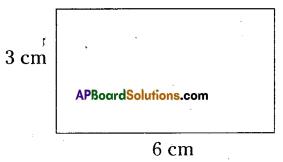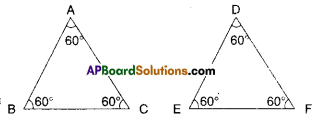AP State Syllabus AP Board 9th Class Maths Solutions Chapter 15 Proofs in Mathematics Ex 15.4 Textbook Questions and Answers.

## AP State Syllabus 9th Class Maths Solutions 15th Lesson Proofs in Mathematics Exercise 15.4Question 1.
State which of the following are mathematical statements and which are not ? Give reason.
i) She has blue eyes.
Solution:
This is not a mathematical statement. no mathematics is involved in it.

ii) x + 7 = 18
Solution:
This is not a statement, as its truthness cant be determined.

iii) Today is not Sunday.
Solution:
This is not a statement. This is an am biguous open sentence.

iv) For each counting number x, x + 0 = x.
Solution:
This is a mathematical statement.v) What tune is it?
Solution:
This is not a riathematical statement.

Question 2.
Find counter examples to disprove the following statements.
i) Every rectangle is a square.
Solution:A rectangle and square are equiangular i.e., all the four angles are right angles. This doesn’t mean that they have equal sides.

ii) For any integers x and y,
$$\sqrt{x^{2}+y^{2}}$$ = x + y
Solution:
Let x = 3; y = 8
$$\sqrt{x^{2}+y^{2}}=\sqrt{3^{2}+8^{2}}$$
= $$\sqrt{9+64}=\sqrt{73}$$

x + y = 3 + 8 = 11
Here, √73 ≠ 11
i.e., $$\sqrt{x^{2}+y^{2}}$$ ≠ x + y

iii) If n is a whole number then 2n2 +11 is a prime.
Solution:
If n = 11 then 2n2+ 11 = 2 (11)2 + 11
= 11 (2 × 11 + 1) = 11 × (22 + 1)
= 11 × 23 is not a prime.iv) Two triangles are congruent if all their corresponding angles are equal.
Solution:If the corresponding angles are equal then the triangles are only similar.

v) A quadrilateral with all sides are equal is a square.
Solution:
A rhombus is not a square, but all its sides are equal.

Question 3.
Prove that the sum of two odd numbers is even.
Solution:

 Steps Reasons 1) (2m + 1); (2n + 1) be the two odd numbers General form of an odd number. 2) (2m + 1) + (2n + 1) = (2m + 2n + 2) = 2 (m + n + 1) = 2K Hence proved. Adding the two numbers General form of an even number.Question 4.
Prove that the product of two even numbers is an even number.
Solution:

 Steps Reasons 1) Let 2m and 2n be two even numbers. General form of an even number. 2) 2m.2n = 4mn = 2(2mn) = 2K Taking the product Rearranging the numbers. 3) 2K where K = 2mn K=2mn 4) Even number Hence proved. General form of an even number.

Question 5.
Prove that if x is odd, then x2 is also odd.
Solution:
Let x be an odd number.
Then x = 2m + 1
(general form of ah odd number) Squaring on both sides,
x2 = (2m + 1)2
= 4m2 + 4m +1
= 2 (2m2 + 2m) + 1
= 2K + 1 where K = 2m2+ 2
Hence x2 is also odd.Question 6.
Examine why they work ?
Solution:
Choose a number = x say
Double it = 2x
Add nine = 2x + 9
= 2x + 9 + x = 3x + 9
Divide by 3 = (3x + 9) ÷ 3
= $$\frac{3 x}{3}+\frac{9}{3}$$ = x + 3
Add 4 ⇒ x + 3 + 4 = x + 7